{{ message }}

# franciscop / ola Public

🌊 Smooth animation library for interpolating numbers

Switch branches/tags
Nothing to show

## Files

Failed to load latest commit information.
Type
Name
Commit time

# OlaSmooth animation library for inbetweening / interpolating numbers in realtime: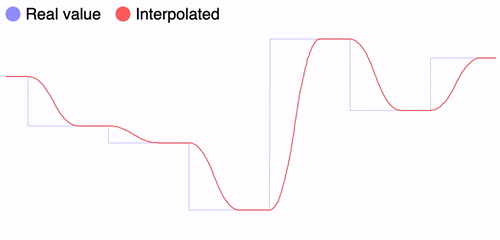```// Start tracking the value
const pos = Ola({ y: 0 });

// Set the value to update async
pos.set({ y: 100 });

// Read the evolution over time
setInterval(() => graph(pos.y), 5);```

It works with multiple values/dimensions: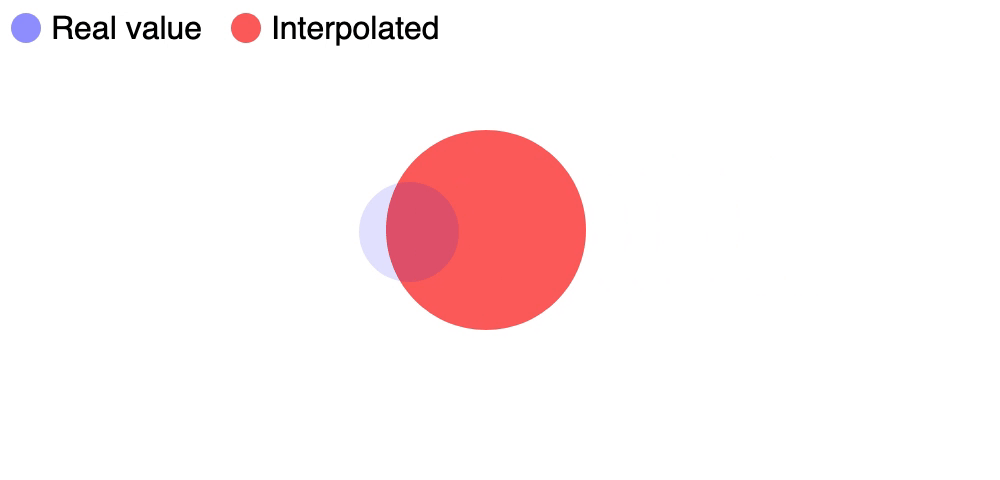```const pos = Ola({ x: 0, y: 0 });

pos.set({ x: e.pageX, y: e.pageY });
});

setInterval(() => {
ball.style.left = `\${pos.x}px`;
ball.style.top = `\${pos.y}px`;
}, 10);```

Also works great with many instances since they are independent: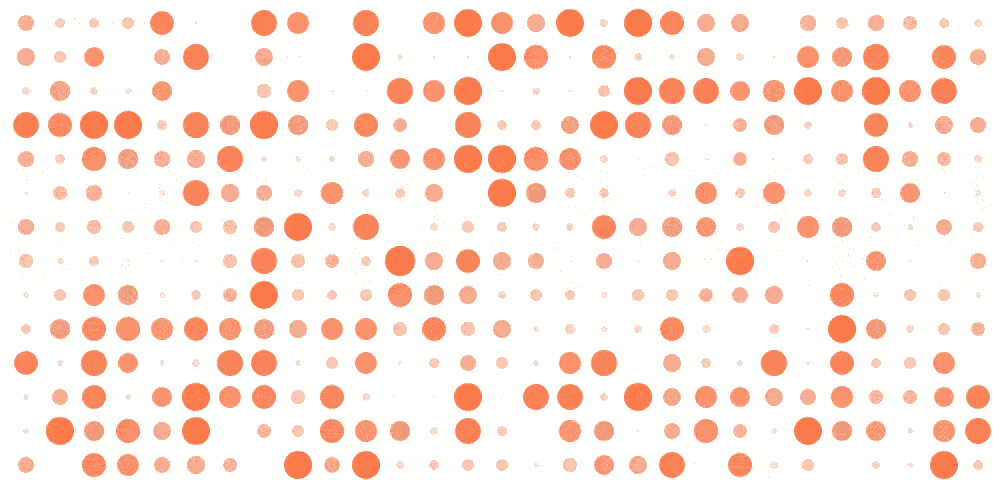```// Generates 1000 instances seamlessly
const dots = Ola(Array(1000).fill(0));

setInterval(() => dots.forEach((dot, i) => {
dots[i] = Math.random();
}), 600);

// ... read + paint screen here```

Tip: click on the GIFs for a live demo with the code :)

## Getting started

Install it with npm:

``````npm install ola
``````

Then import it and use it:

```import Ola from "ola";
const pos = Ola({ x: 0 });
console.log(pos.x); // 0```

If you prefer to use a CDN:

```<script src="https://cdn.jsdelivr.net/npm/ola"></script>
<script type="text/javascript">
const pos = Ola({ x: 0 });
console.log(pos.x); // 0
</script>```

## Documentation

There are three distinct operations that can be run: creating an instance, setting it to update and reading it.

### Create an instance

`Ola(initial, time = 300);`

The first parameter is the initial value. It can be either a single number, or an object of `key:numbers` or an array of numbers:

```const heater = Ola(20); // Alias of `{ value: 20 }`
const motor = Ola({ angle: 180 }); // A named parameter for clarity
const position = Ola({ x: 0, y: 0 }); // Any number of properties
const heights = Ola([0, 0, 0, 0]); // A group of heights```

The second parameter is how long the transition will last. It should be a number that represents the time in milliseconds:

```const heater = Ola(20); // Default = 300 ms
const motor = Ola({ angle: 180 }, 1000); // Turn the motor slowly
const position = Ola({ x: 0, y: 0 }, 100); // Quick movements for the position
const heights = Ola([0, 0, 0, 0], 300); // 300, same as the default```

Passing a single number as a parameter is the same as passing `{ value: num }`, we are just helping by setting a shortname. It is offered for convenience, but recommend not mixing both styles in the same project.

It works with Javascript numbers, but please keep things reasonable (under `Number.MAX_VALUE / 10`):

```console.log(Ola(100));
console.log(Ola(-100));
console.log(Ola(0.001));
console.log(Ola(1 / 100));```

The time it takes to update can also be updated while setting the value, which will update it for any subsequent transition:

```// All `pos.set()` will take 1 full second
const pos = Ola({ x: 0 }, 1000);
pos.set({ x: 100 }, 3000);```

### Update the value

```heater.value = 25; // Since the constructor used a number, use `.value`
motor.angle = 90; // Turn -90 degrees from before
position.set({ x: 100, y: 100 }); // Move 0,0 => 100,100
heights = 120; // Move the second (0-index) item to 120```

When we update a property it is not updated instantaneously (that's the whole point of this library), but instead it's set to update asynchronously:

```const pos = Ola({ x: 0 });
pos.set({ x: 100 });

// 0 - still hasn't updated
console.log(pos.x);

// 100 - after 300ms it's fully updated
setTimeout(() => console.log(pos.x), 1000);```

Remember that if you set the value as `Ola(10)`, this is really an alias for `Ola({ value: 10 })`, so use the property `.value` to update it:

```heater.value = 25;
heater.set({ value: 25 });```

You can see in this graph, the blue line is the value that is set though `.set()`, while the red line is the value that reading it returns:```log(heater.value); // Since the constructor used a number, use `.value`
log(motor.angle); // Read as an object property
log(position.get("x")); // Find the X value
log(heights); // Move the first item to 120```

You can read the value at any time, and the value will be calculated at that moment in time:

```const pos = Ola({ x: 0 });
pos.set({ x: 100 });

setInterval(() => {
// It will update every time it's read
console.log(pos.x);
}, 10);```

In contrast to other libraries, there's no need to tick/update the function every N ms or before reading the value, since `Ola()` uses math functions you should just read it when needed.

If you need to access more advanced features, you can read these two properties:

```// All the details about the current transition, please see the source for more info
log(heater._value);  // { to: 25, from: 20, ... }
log(motor._angle);  // { to: 90, from: 180, ... }

// The value that will be set when the transition is finished
log(heater.\$value);  // 25
log(motor.\$angle);  // 90```

## Features

While there are some other great libraries like Tween, this one has some improvements:

### Smooth in realtime

Other libraries don't move smoothly when there's an update while the previous transition is still ongoing. Ola makes sure there are no harsh corners: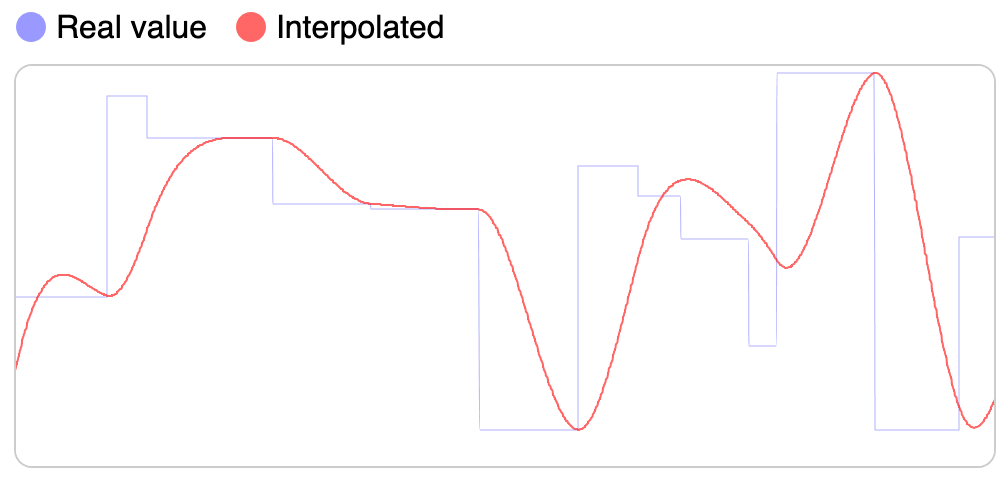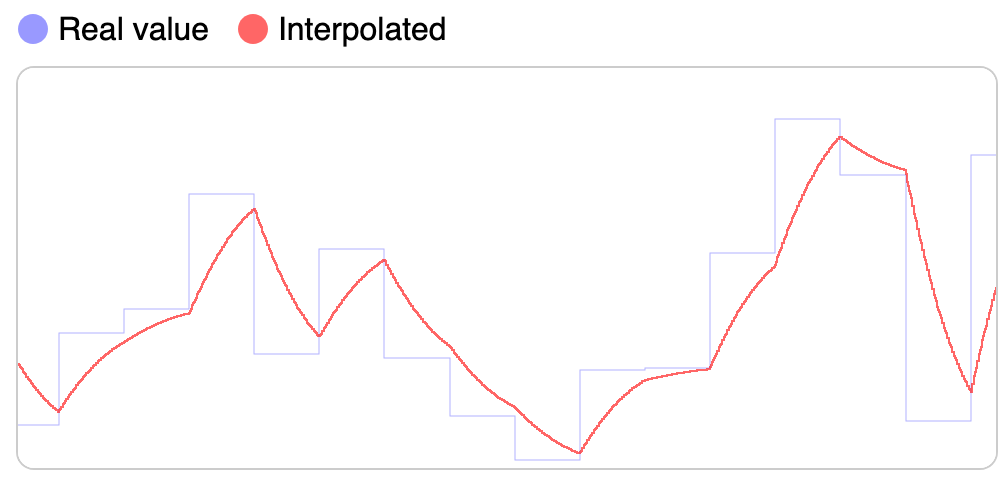Smooth interpolation with Ola() Harsh interpolation with Tweenmax

Status of libraries updating animation mid-way:

Since this is driven by mathematical equations, the library doesn't calculate any value until it needs to be read/updated. It will also only change the one we need instead of all of the values:

```const position = Ola({ x: 0, y: 0 });
position.x = 10; // Only updates X
console.log(position.x); // Calculates only X position, not y```

Not only this is great for performance, but it also makes for a clean self-contained API where each instance is independent and portable.

## Others from Author

Like this project? Francisco has many more! Check them out:

• server.js - a batteries-included Node.js server
• translate.js - to easily translate text on the browser and Node.js